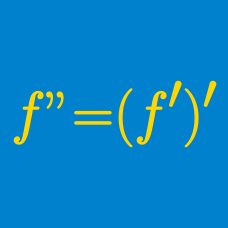Calculus

# Graphical Interpretation of Higher Order Derivatives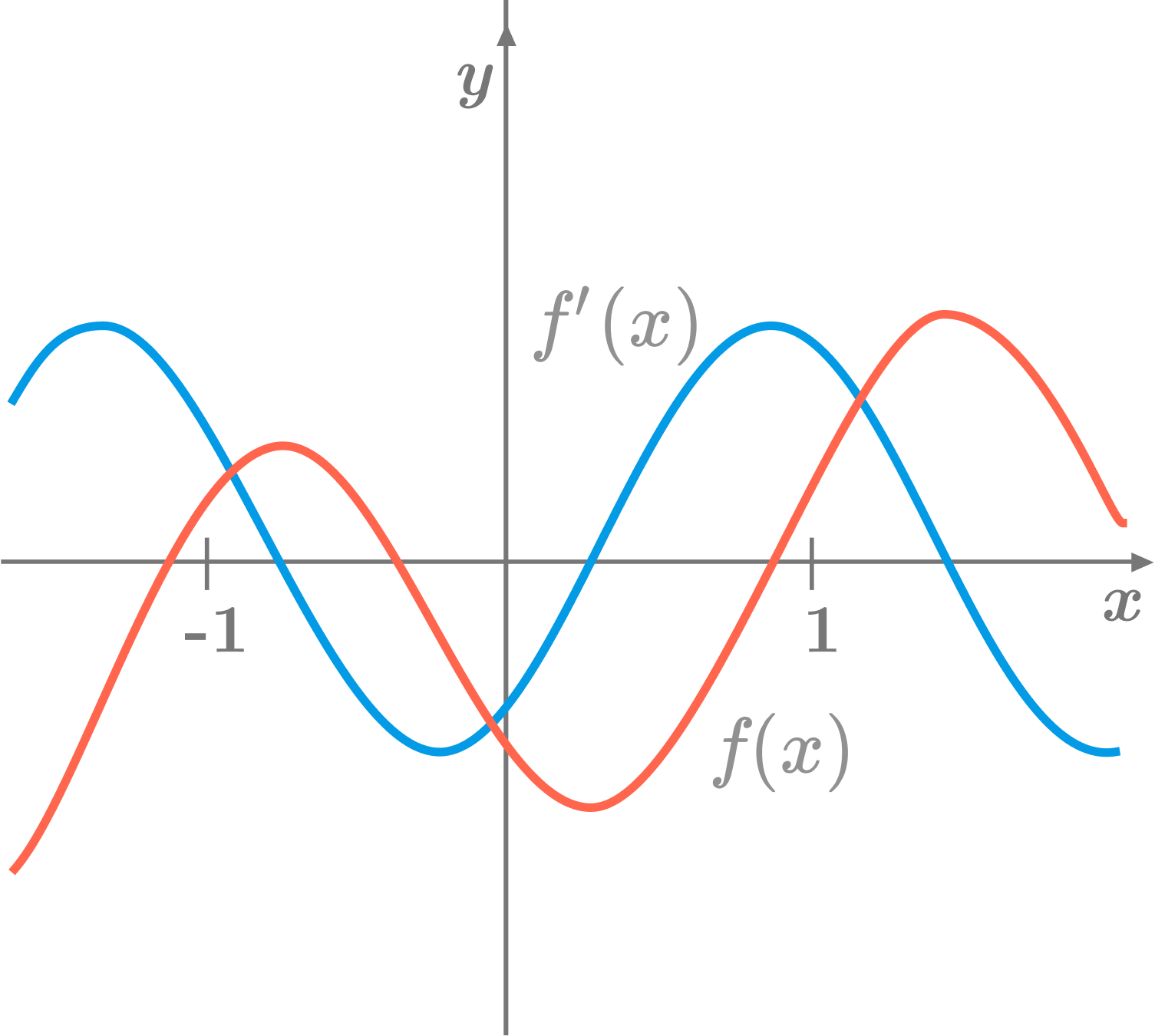In the above diagram, the red curve is the graph of a function $f,$ and the blue curve is the graph of its first derivative $f'.$ What is the relationship between $f'(-1)$ and $f''(1) ?$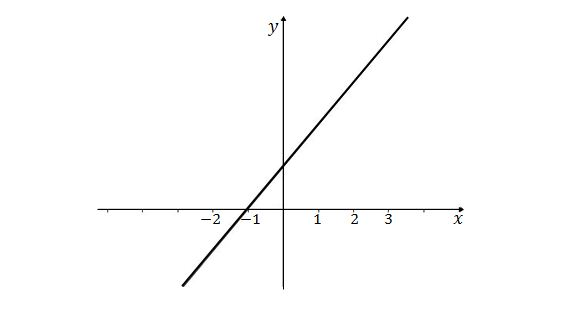Given the graph of $y=f''(x)$ above, which of the following is a possible graph of $y=f(x)?$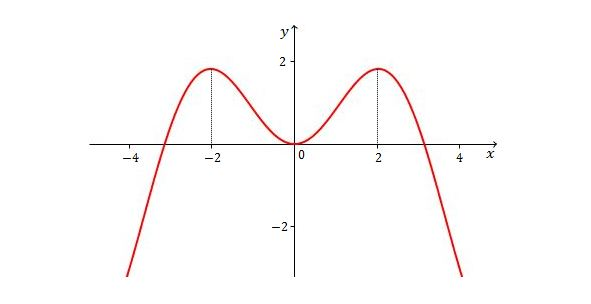Given the graph of $y=f(x)$ above, which of the following is a possible graph of $y=f''(x)?$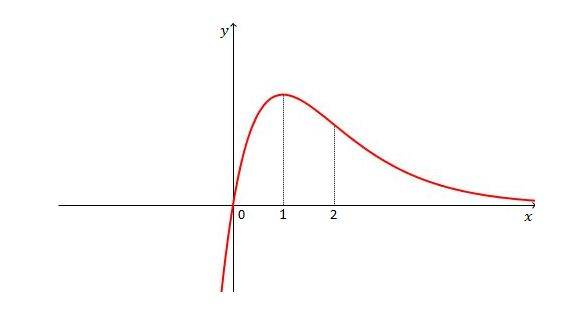Given the graph of $y=f(x)$ above, which of the following is a possible graph of $y=f''(x)?$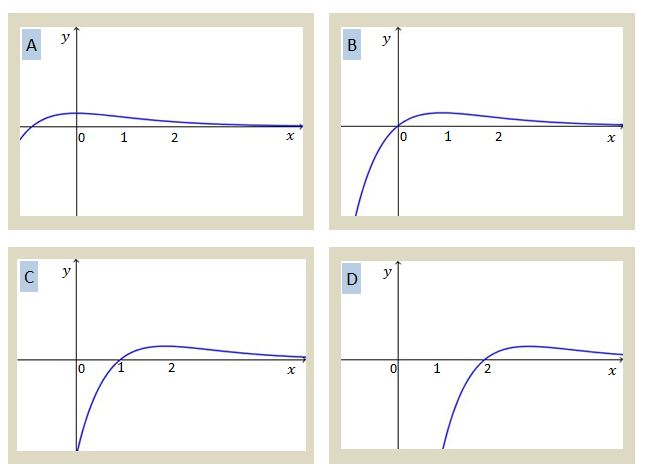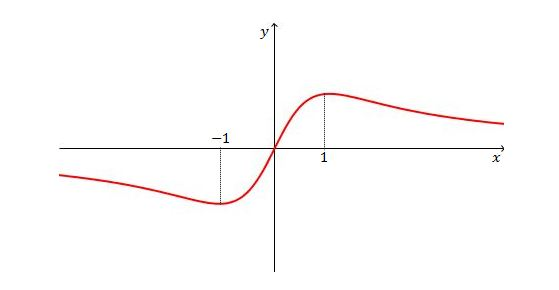Given the graph of $y=f(x)$ above, which of the following is a possible graph of $y=f''(x)?$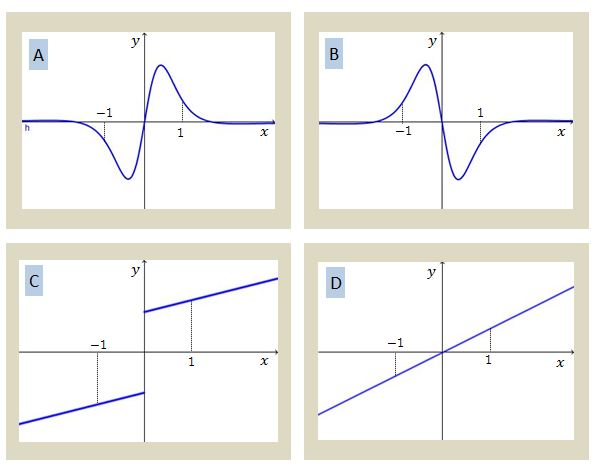×